# Long Division Can Be EasyPage 1

#### WATCH ALL SLIDES

Slide 1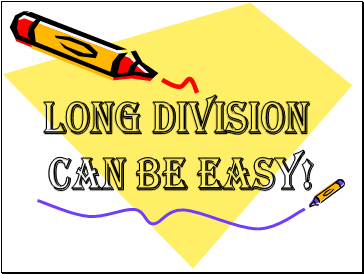LONG DIVISION

CAN BE EASY!

Slide 2## Long Division

L.O - To know how to use DMSB to work out long division.

R.T-

(Remember To)

Divide

Multiply

Subtract

Bring Down

Slide 3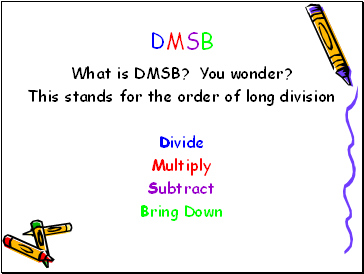## DMSB

What is DMSB? You wonder?

This stands for the order of long division

Divide

Multiply

Subtract

Bring Down

Slide 4Long Division

Take a look at this division problem:

3 )74

Looks scary, huh? Well, when you use DMSB It can be simple. Move to the next slide to get started!

This is the dividend. The dividend is the number we break into groups.

This is the divisor. The divisor is the number of groups we are dividing into or the number we are dividing by.

Slide 5## Divide

First, we want to DIVIDE.

Ask yourself: “How many times can 3 go into 7?”

3 )74

Slide 6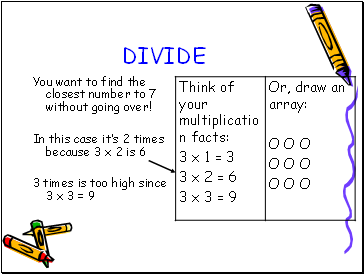DIVIDE

You want to find the closest number to 7 without going over!

In this case it’s 2 times because 3 x 2 is 6

3 times is too high since 3 x 3 = 9

Slide 7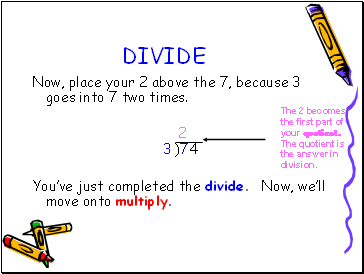DIVIDE

Now, place your 2 above the 7, because 3 goes into 7 two times.

2

3 )74

You’ve just completed the divide. Now, we’ll move onto multiply.

The 2 becomes the first part of your quotient. The quotient is the answer in division.

Slide 8## Multiply

Next, you want to multiply 3 times 2:

2

3 )74

3 x 2= 6 6

We do this because we said that 3 can go into 7 two times, but now we have to find out how close to 7 we can get. So we multiply!

Slide 9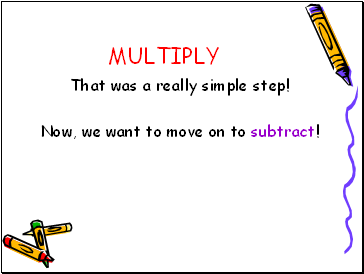MULTIPLY

That was a really simple step!

Now, we want to move on to subtract!

Slide 10## Subtract

Now that we know how many times 3 goes into 7, we have to subtract to see the difference between the numbers:

2

3 )74

-6

1

Good! We know 7 – 6 = 1. Leave your 1 in it’s spot, because we’re going to use it for the bring down!

Slide 11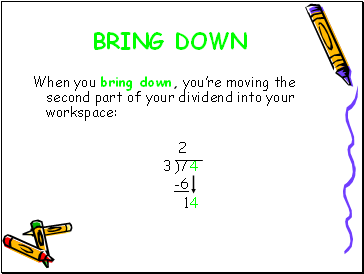## Bring down

When you bring down, you’re moving the second part of your dividend into your workspace:

Go to page:
1  2  3